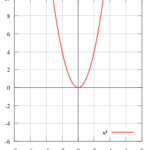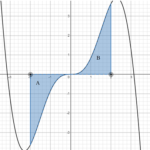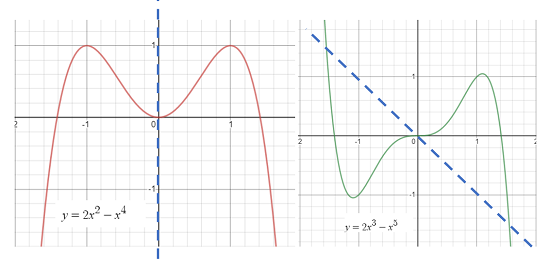# Symmetry of a Function: Testing For

Contents:

## What is a Symmetrical Function?

“Symmetry of a Function” usually refers to symmetry of a function’s graph. Even and odd functions are symmetrical:

• Even functions are symmetrical about the vertical y-axis. The graph on the right-hand side (quadrant 1) is a mirror image of the graph on the left-hand side (quadrant 2).An even function is symmetrical along the vertical axis.
• Odd functions are symmetric about the origin. This is defined mathematically as f(-x) = x for every x in the domain.Graph of a symmetrical odd function.

There are some specific polynomial functions that are called “symmetric” not because their graph is symmetrical, but because the polynomials remain the same if you permute their roots. See: Symmetric Polynomial Functions

## What is the Axis of Symmetry?The function on the left is symmetrical to the y-axis; The function on the right is symmetric to the origin. The blue dashed line is the axis of symmetry.

The axis of symmetry (also called the line of symmetry) is a line that creates two sides: each side is a mirror image.

For parabolas, the axis of symmetry is a vertical line drawn through the vertex (the highest or lowest point of the graph). The equation for the axis of symmetry is the x-value of the vertex coordinates. For example, if the vertex of a parabola is (1 , 2), the formula for the axis of symmetry is x = 1.

## Center of Symmetry

Two points are symmetrical with respect to a center of symmetry (a point) if they are on the same line and are an equal distance from the center of symmetry. The two points are related to each other by a 180° turn.## How to Test for Symmetry of a Function

A useful fact about polynomials is that they are symmetric with respect to the y-axis when every term is either a constant or has an even exponent. For other functions, you could just graph them to test for symmetry. However, it may not be easy to see symmetry on a graph. For example, the following graph is symmetric around the origin, but it’s challenging to see that because of the wild oscillations:A better way is to test for symmetry of a function using a little algebra. All you have to do is work your way down the list of three possibilities:

• Replace x by -x. If you get the same function, then that function is symmetric over the y-axis.
• Replace y by -y. If you get the same function, then that function is symmetric over the x-axis.
• Replace x by -x and y by -y. If you get the same function, then that function is symmetric with respect to the origin.

Example question: Is y = 2x3 – x symmetric?

Solution:

• Replace x by -x and then simplify:
• y = 2x3 – x →
• y = 2(-x)3 – (-x) →
• y = 2x3 + x

This gives a different function, so y = 2x3 – x is not symmetric to the y-axis.

• Replace y by -y.
• y = 2x3 – x →
• -y = 2x3 – x

This also gives a different function, so y = 2x3 – x is not symmetric to the x-axis either.

• Replace x by -x and y by -y.
• y = 2x3 – x →
• Replacing x and y: -y = 2(-x)3 -(- x) →
• Simplifying: -y = -2x3 + x→
• Multiply by -1: y = 2x3

This results in the same function, so y = 2x3 – x is symmetric around the origin.

## References

Larson, R. & Edwards, B. (2018). Calculus: Early Transcendental Functions. Cengage Learning.
Graphs drawn with Desmos.

CITE THIS AS:
Stephanie Glen. "Symmetry of a Function: Testing For" From StatisticsHowTo.com: Elementary Statistics for the rest of us! https://www.statisticshowto.com/symmetry-of-a-function/